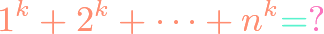# Algebra## Polynomial Integer Root Theorem

https://www.youtube.com/watch?v=fgBBJnhbMvI There are a couple of related theorems that we interchangeably call the “integer root theorem.” One tells us how to find that integer roots of a polynomial with integer coefficients. The second tells us that all rational roots of a monic polynomial with integer coefficients are integers. We prove both results in this video.https://www.youtube.com/watch?v=t9RuFHcyQRc&t=60s The ordinary way of proving the quadratic formula $$x=frac{-bpmsqrt{b^2-4ac}}{2a}$$ is through a process called “completing the square.” But there is one step at the end which requires casework that almost everyone glosses over. In this video, we show a little-known modificiation of completing the square that avoids the need for any casework.